# Cost of Equity

The rate of return shareholders expect for investing in equity

The cost of equity measures the return that shareholders expect from their investments. Companies use it as part of internal investment decisions. It is also used when deciding external acquisition opportunities. The traditional models for calculating the cost of equity are the dividend capitalization and the capital asset pricing model (CAPM).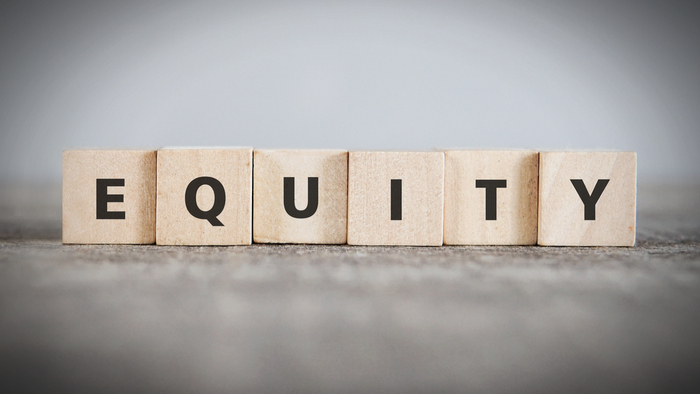The cost of equity, when combined with the cost of debt as part of WACC, reflects the rate of return that companies are required to generate on their investments. Therefore, the WACC is compared with the expected return of an investment. If the WACC is higher than the profit on investment, then the investment will be acceptable from stakeholders' point of view and vice versa.
The dividend capitalization model can only be used for companies that issue dividends. On the other hand, the CAPM formula can be applied to all publicly traded companies.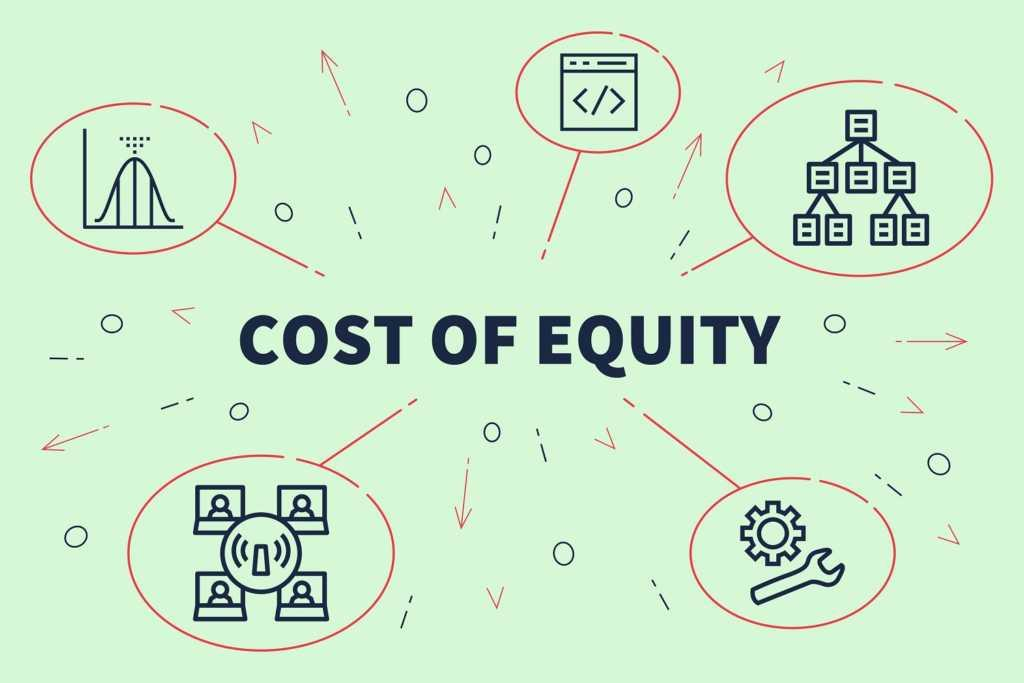## Dividend Capitalization Model

The dividend capitalization model can only be used with publicly traded companies that issue dividends. It is much simpler when compared to the CAPM model as it relies on Below is the formula for the dividend capitalization: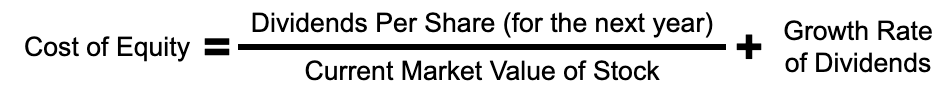The dividend capitalization formula consists of three parts. Here is a breakdown of each part:

• Dividends Per Share - The first is determining the expected dividend for the next year. Management should have a good estimate of the expected dividend for the upcoming year. The best way to estimate the expected dividend is to look at the past rates of dividend as well as its growth rate. Another factor that can help estimate the expected dividend is the company's forecasted earnings.
• Current Market Value of Stock - The divisor in the formula is the stock's market value. This is simple and can be picked up from the stock market quotes.
• Growth Rate of Dividends - The final part of the equation is the growth rate of dividends. Just as we relied on historical data to calculate the expected dividend, we can use the same historical data to calculate the growth rate.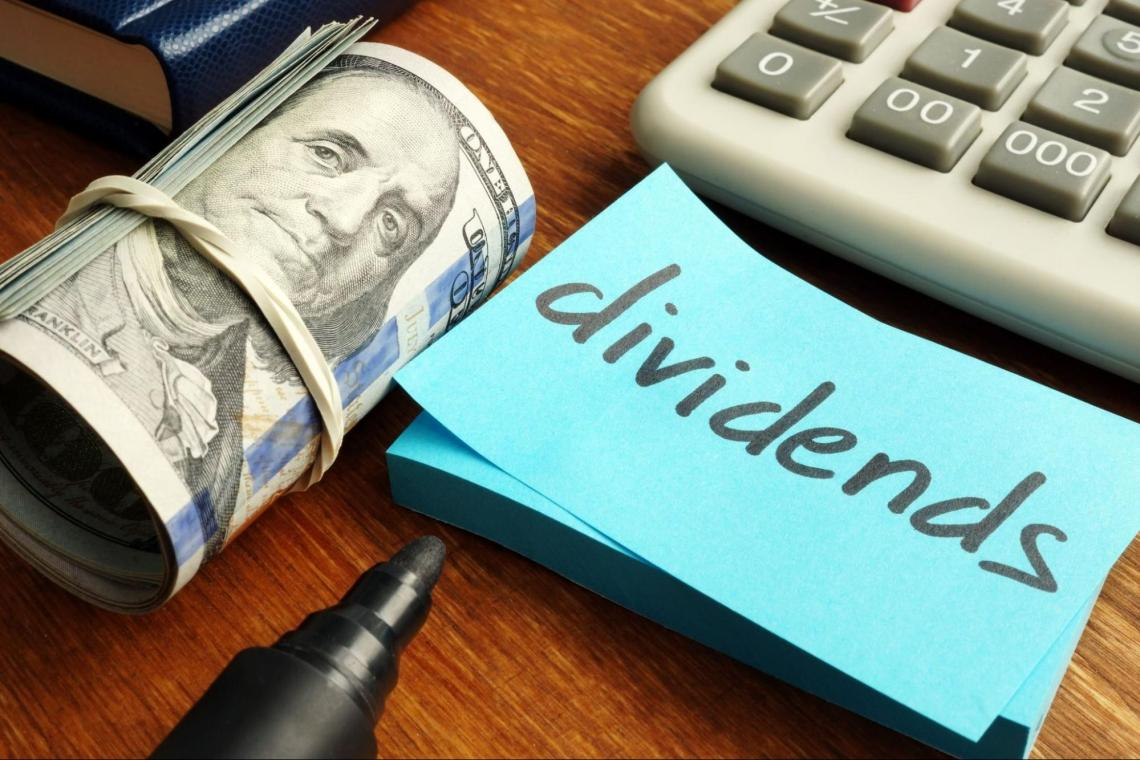## Example of Dividend Capitalization

Company X is a large nationwide retailer. Their most recent share price was \$250, and they announced an annual dividend of \$5. Last year, their annual dividend was \$4.50, and the year before was \$4.00. This year the company earnings per share were \$8, and it is expected that next year's earnings per share will be \$10.

To find the expected dividend for next year, we must find the dividend payout ratio from this year. The company earned \$8 per share and paid out \$5 in dividends. The payout ratio would be 8/5 or 0.625. So if next year's earnings per share are \$10, we must multiply that amount by 0.625, which gives us \$6.25 as the expected dividend payment for next year.

To find the market value of the share price, we can look at the most recent share price. The most recent share price was \$250. So, the current market value of a stock is \$250.

Finally, finding the growth rate of dividends is based on historical dividend payment. For example, the increase in dividend payment during the previous two years was 12.5% and 11.1%, respectively. This means that the average dividend growth rate would be 11.8%.

Putting the three values in the cost of equity formula, we get:

Cost of equity = (6.25/250) + 0.118

= 0.026 + 0.118

= 0.144 or 14.4%

## Capital Asset Pricing Model (CAPM)

The capital asset pricing model (CAPM) is another method to determine the cost of equity for public companies. Unlike the dividend capitalization formula, this model doesn't rely on dividends to determine the cost and hence can be used with companies that don't distribute dividends. However, this model is more reliant on assumptions than actuals and is more prone to manipulation.

The formula for CAPM is given below:The capital asset pricing model formula comprises three parts. Here is a breakdown of each part:

• Risk-Free Rate of Return - The risk-free rate is the rate of return one can expect to generate on investment with zero risk. While no investment carries zero risk, most investors agree that US treasury bonds carry the least risk across all assets. To find the risk-free rate, look up the yields on the treasury.
• Beta - This measures how much a company's stock price is expected to rise or fall in response to market changes. It is calculated by comparing the company's stock return to that of the market as a whole over a certain period and then calculating the correlation coefficient. A beta of 1 means that the stock's past performance is the same as that of the market, which gives it a low volatility rating. A beta higher than one means its price is more volatile than the market.
• Market Rate of Return - This is the expected return of an investment in the general stock market. The most commonly used standard of market returns is the S&P 500. On average, the S&P has been growing at about 10% annually.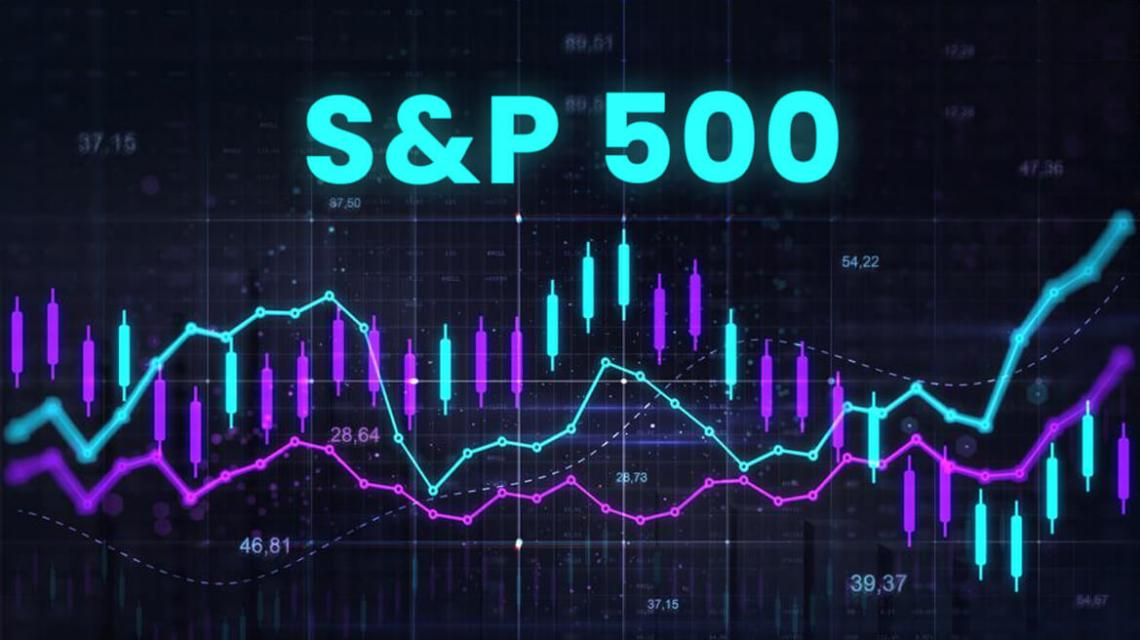## Example of CAPM

Company Y is a technology company that is still breaking into the industry and has a beta of 1.25. The current market inflation rate is 4%. The US treasury bill rate is 1.5%. Finally, the S&P 500 is expected to keep pace with historical growth of 10%.

The risk-free rate can be determined by looking at the treasury rate, which is 1.5%. The other values are given directly, so we can plug them directly into the formula to find the cost of equity (Ke).

Ke = 1.5% + 1.25 * (10 - 1.5%) = 12.125%

## What is Equity?

Owning equity in a company means you have ownership within the company. It also means that in case of liquidation, the equity holders will get their funds after every other stakeholder has been paid.

Equity is also referred to as shareholders' equity. The value of shareholders' equity can be found on a company's balance sheet. To find the total equity shareholders own, you must subtract the total assets by total liabilities. This value will be the amount of shareholder equity.

The value of equity is essential to investors because it helps them measure the size of their stake in a company in monetary terms. In addition, it gives investors the ability to profit from company earnings in the form of dividends and vote on actions within the company.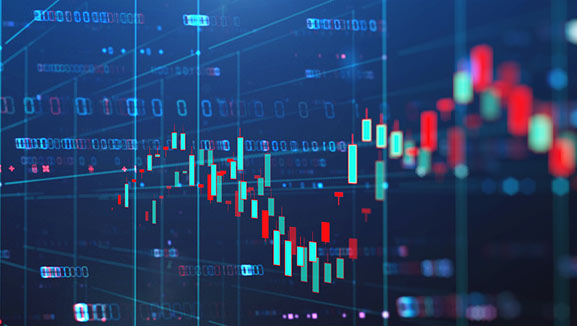## What the Cost of Equity Tells You

The cost of equity can be helpful for companies that are looking to raise funds. For example, a company with a low cost of equity should be able to raise equity rather easily than one with a high cost of equity.

Companies also use the cost of equity when deciding on acquisitions. Given the acquisition cost, companies will look at the target company's equity cost to determine if the risk is acceptable or not.## Dividend Capitalization vs. CAPM

While both the dividend capitalization and capital asset pricing model are used to find the cost of equity, the equations are very different. For starters, the dividend capitalization model can only be used by companies that offer dividends, while the capital asset pricing model can be used for any public company

The dividend capitalization formula is easier to forecast than CAPM as it relies on factual data. Hence, it can be argued that it is the more accurate among the two. On the other hand, CAPM relies on assumptions, making it harder and more prone to errors.

Both formulas can be used to find the cost of equity. However, it is essential to note the use cases and limitations of both.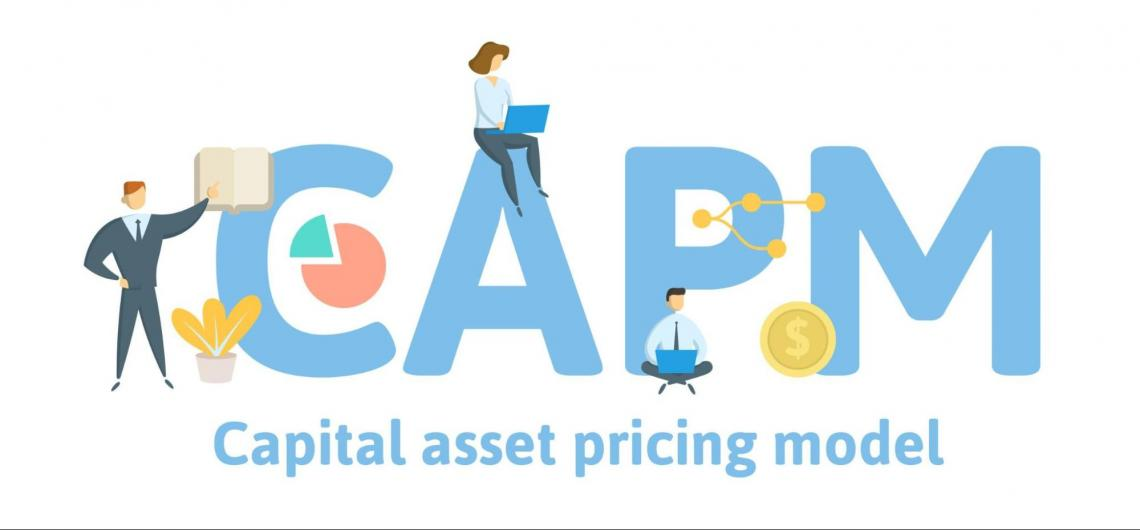## Cost of Equity vs. Cost of Debt vs. Cost of Capital vs. WACC

The cost of debt - Is the effective rate that a company pays on debt instruments. It can be calculated before and after taxes depending on the context for use. For example, it is most commonly used when companies decide to issue bonds or take loans. Simply put, the cost of a company's debt is the average interest rate on all its debts. Companies that have a lower cost of debt when compared to the cost of equity tend to prefer raising funds through debt and vice versa.

Cost of capital - Is the overall cost of raising funds to build a new business. It incorporates the costs of both debt and equity funding.

The weighted average cost of capital (WACC) - Is the cost of each source of fund, weighted by their proportion in the capital structure. WACC is the most commonly used method of determining the cost of raising funds due to its prominence in the discounted cash flow valuation method

## Cost of Equity Calculator

The cost of equity calculator is an online tool that calculates the cost of equity. If you are looking to calculate the cost of equity, using this calculator quickly will help. However, these calculators require you to input the necessary values in the formula.

Omni Calculator has a free and easy-to-use cost of equity calculator. You can select from using both the dividend capitalization and capital asset pricing model. Then plug in the values, and the calculator does the rest. Here is a snapshot of the Omni Calculator cost of equity calculator.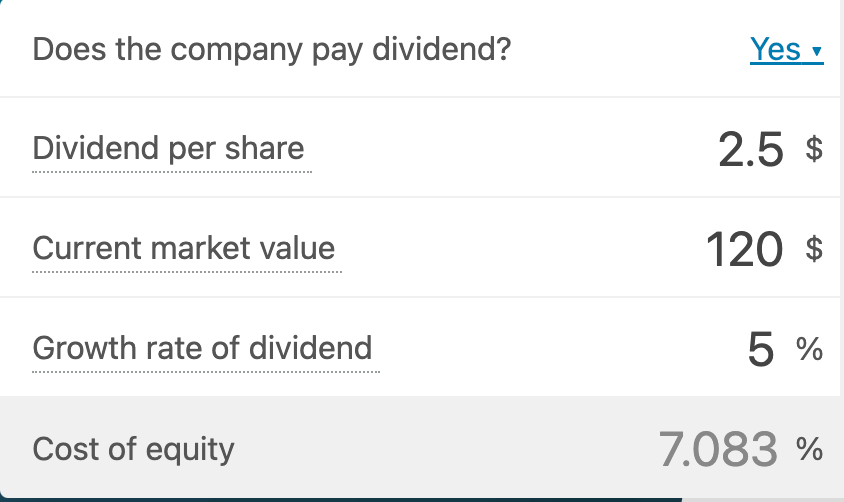### Everything You Need To Master Excel Modeling

To Help You Thrive in the Most Prestigious Jobs on Wall Street.